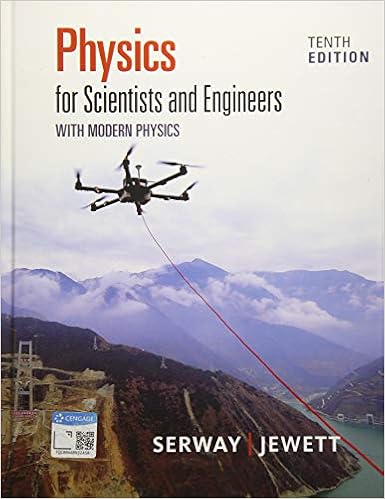# Lab Experiment #3.doc - Experiment#3 The Addition and...

• Lab Report
• 8

This preview shows page 1 - 7 out of 8 pages.

##### We have textbook solutions for you!
The document you are viewing contains questions related to this textbook.The document you are viewing contains questions related to this textbook.
Chapter 7 / Exercise 7.3
Physics for Scientists and Engineers with Modern Physics
Jewett/SerwayExpert Verified
Experiment #3The Addition and Resolution of Vectors: The Force TablePHY-186-002LSby: David SotoLab Partners:#1 Asma Alyovsef #2 Renato Hernandez#3 Kinjal PatelDate: 2/28/19
##### We have textbook solutions for you!
The document you are viewing contains questions related to this textbook.The document you are viewing contains questions related to this textbook.
Chapter 7 / Exercise 7.3
Physics for Scientists and Engineers with Modern Physics
Jewett/SerwayExpert Verified
ALL ORIGINAL data sheet(s) from the experiment manual (follows the Objective).
Data: 2/28/19Formulas:formulauseunitFX=F COS To calculate X componentgFY=F SIN To calculate Y componentgR= Root square (R2+ R2)To calculate the resultant NTan = RY/RXTo determine the angleDegreeDiscussion of Results:a.Analysis of Data and Results–We analyzed the results of different methods of vectors addition in the data table. So, we used the information of each force vector F1 and F2, to calculate the resultant. Then we used the same information to graph and to do the experiment with the force table, then compare the information. What we have learned in this experiment is how to add vectors graphically to find a resultant and add vectors analytically. Vectors have magnitude as well as direction, vector quantity is a quantity that has both a magnitude and a direction and thus can be represented with a vector." Two vectors may be added
•••